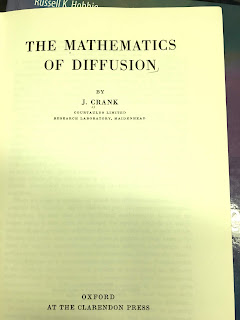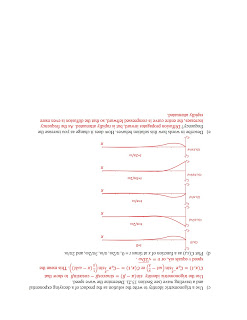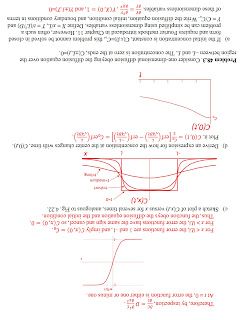## Friday, January 18, 2019

### Five New Homework Problems About Diffusion

Diffusion is a central concept in biological physics, but it's seldom taught in physics classes. Russ Hobbie and I cover diffusion in Chapter 4 of Intermediate Physics for Medicine and Biology.

The one-dimensional diffusion equation,
is one of the “big threepartial differential equations. Few analytical solutions to this equation exist. The best known is the decaying Gaussian (Eq. 4.25 in IPMB). Another corresponds to when the concentration is initially constant for negative values of x and is zero for positive values of x (Eq. 4.75). This solution is written in terms of error functions, which are integrals of the Gaussian (Eq. 4.74). I wonder: are there other simple examples illustrating diffusion? Yes!

In this post, my goal is to present several new homework problems that provide a short course in the mathematics of diffusion. Some extend the solutions already included in IPMB, and some illustrate additional solutions. After reading each new problem, stop and try to solve it!

Section 4.13
Problem 48.1. Consider one-dimensional diffusion, starting with an initial concentration of C(x,0)=Co for x less than 0 and C(x,0)=0 for x greater than 0. The solution is given by Eq. 4.75
where erf is the error function.
(a) Show that for all times the concentration at x=0 is C0/2.
(b) Derive an expression for the flux density, j = -DC/∂x at x = 0. Plot j as a function of time. Interpret what this equation is saying physically. Note:

Problem 48.2. Consider one-dimensional diffusion starting with an initial concentration of C(x,0)=Co for |x| less than L and 0 for |x| greater than L.
(a) Plot C(x,0), analogous to Fig. 4.20.
(b) Show that the solution
obeys both the diffusion equation and the initial condition.
(c) Sketch a plot of C(x,t) versus x for several times, analogous to Fig. 4.22.
(d) Derive an expression for how the concentration at the center changes with time, C(0,t). Plot it.

Problem 48.3. Consider one-dimensional diffusion in the region of x between -L and L. The concentration is zero at the ends, CL,t)=0.
(a) If the initial concentration is constant, C(x,0)=Co, this problem cannot be solved in closed form and requires Fourier series introduced in Chapter 11. However, often such a problem can be simplified using dimensionless variables. Define X = x/L, T = t/(L2/D) and Y = C/Co. Write the diffusion equation, initial condition, and boundary conditions in terms of these dimensionless variables.
(b) Using these dimensionless variables, consider a different initial concentration Y(X,0)=cos(Xπ/2). This problem has an analytical solution (see Problem 25). Show that Y(X,T)=cos(Xπ/2) e2T/4 obeys the diffusion equation as well as the boundary and initial conditions.

Problem 48.4. In spherical coordinates, the diffusion equation (when the concentration depends only on the radial coordinate r) is (Appendix L)

Let C(r,t) = u(r,t)/r. Determine a partial differential equation governing u(r,t). Explain how you can find solutions in spherical coordinates from solutions of analogous one-dimensional problems in Cartesian coordinates.

Problem 48.5. Consider diffusion in one-dimension from x = 0 to ∞. At the origin the concentration oscillates with angular frequency ω, C(0,t) = Co sin(ωt).
(a) Determine the value of λ that ensures the expression
obeys the diffusion equation.
(b) Show that the solution in part (a) obeys the boundary condition at x = 0.
(c) Use a trigonometric identity to write the solution as the product of a decaying exponential and a traveling wave (see Section 13.2). Determine the wave speed.
(d) Plot C(x,t) as a function of x at times t = 0, π/2ω, π/ω, 3π/2ω, and 2π/ω.
(e) Describe in words how this solution behaves. How does it change as you increase the frequency?

Of the five problems, my favorite is the last one; be sure to try it. But all the problems provide valuable insight. That’s why we include problems in IPMB, and why you should do them. I have included the solutions to these problems at the bottom of this post (upside down, making it more difficult to check my solutions without you trying to solve the problems first).Random Walks in Biology,by Howard Berg.
Interested in learning more about diffusion? I suggest starting with Howard Berg’s book Random Walks in Biology. It is at a level similar to Intermediate Physics for Medicine and Biology.

After you have mastered it, move on to the classic texts by Crank (The Mathematics of Diffusion) and Carslaw and Jaeger (Conduction of Heat in Solids). These books are technical and contain little or no biology. Mathephobes may not care for them. But if you’re trying to solve a tricky diffusion problem, they are the place to go.

Enjoy!The Mathematics of Diffusion.The Conduction of Heat in Solids.I told you these books are technical! (Page 45 of Crank)Page 4Page 3Page 2Page 1